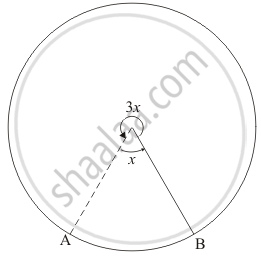Advertisement Remove all ads

# In a circle, the major arc is 3 times the minor arc. The corresponding central angles and the degree measures of two arcs are - Mathematics

MCQ

In a circle, the major arc is 3 times the minor arc. The corresponding central angles and the degree measures of two arcs are

#### Options

•  90° and 270°

• 90° and 90°

•  270° and 90°

• 60° and 210°

Advertisement Remove all ads

#### Solution

270° and 90°

We are given the major arc is 3 times the minor arc. We are asked to find the corresponding central angle.

See the corresponding figure.We know that angle formed by the circumference at the centre is 360°.

Since the circumference of the circle is divided into two parts such that the angle formed by major and minor arcs at the centre are 3x and respectively.

So             3x + x = 360

4x = 360

x = 90

So m $\stackrel\frown{AB}$ = 90° and m $\stackrel\frown{AB}$   = 3x = 270°

Is there an error in this question or solution?
Advertisement Remove all ads

#### APPEARS IN

RD Sharma Mathematics for Class 9
Chapter 15 Circles
Q 10 | Page 110
Advertisement Remove all ads

#### Video TutorialsVIEW ALL 

Advertisement Remove all ads
Share
Notifications

View all notifications

Forgot password?
Course